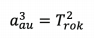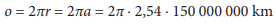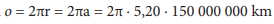# Practical Exercise 2: Minor Planet Velocity

Practical Exercise: The minor planet marked (5000) IAU is located at a distance of 2.54 au from the Sun. Assume a circular orbital trajectory. What is its orbital period in seconds?

Answer: Assuming a circular orbital trajectory, the principal half-axis is equal to a = 2,54 au. We work with the Kepler's third law (law of periods), where the following applies to objects orbiting the Sun:By substitution we find out T = 4,05 roka = 1,28 ∙ 108 s.

The resulting orbital period is obtained by multiplying the value of the principal half-axis in astronomical units to the third (a3 = a ∙ a ∙ a) and finding the square root of the result (√).

Practical Exercise: Estimate the velocity of a minor planet on an orbiting trajectory around the Sun, assuming that the trajectory of the minor planet around the Sun is circular.

Answer: The average velocity of the minor planet is calculated v  = s  / T. Assume that 1 au = 150 000 000 km. We need to calculate the trajectory of the orbit using a formula= 2,39 bilion km.

v = s / T = 2,39 ∙ 109 : 1,28 ∙ 108 km · s–1 = 18,7 km · s–1

Practical Exercise: How would the orbital velocity of the minor planet change if it were at a distance of the planet Jupiter?

Answer: The average distance to Jupiter is 5.20 au. The orbital period according to Kepler's third law is 11,9 years = 3,75 ∙ 108 s. The average velocity of the minor planet is calculated v = s / T. Let’s assume that 1 au = 150 000 000 km. We need to calculate the trajectory of the orbit using a formula= 4,90 miliardy km.

v = s / T = 4,90 ∙ 109 : 3,75 ∙ 108 km · s–1 = 13,1 km · s–1